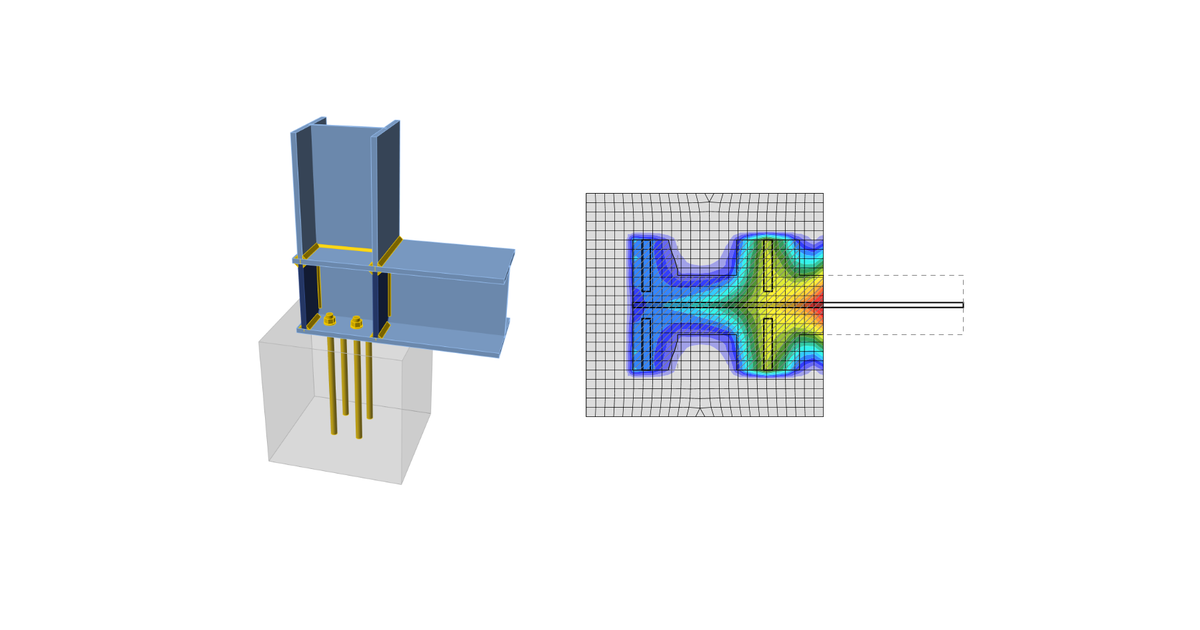### Choose language# Code-check of concrete block according to Hong Kong Code

$$Concrete in bearingConcrete in bearing is checked according to CoP – SUoS – Cl. 9.4.1 as:$\sigma \le w$where: $$\sigma$$ – average compressive stress at effective area $$A_{eff}$$ which is the intersection of two areas: $$A_{CM}$$ – effective area determined according to Cl. 9.4.1 for pure compression $$A_{FEM}$$ – area under the base plate in contact with concrete determined by FEA $$w = 0.6 f_{cu}$$ – concrete compressive resistance against concentrated load $$f_{cu}$$ – concrete minimum characteristic compressive strength Effective area $$A_{CM}$$ is the area of steel member including stiffeners welded to the base plate increased by overlap $$c$$:$c = t_p \sqrt{\frac{p_{yp}}{3w}}$where: $$t_p$$ – base plate thickness $$p_{yp}$$ – design yield strength of the base plate The pressure under the compression zone is considered as uniform.Transfer of shearThe shear action at the base plate is assumed to be transferred from the column to the concrete foundation by: Friction between base plate and concrete/grout Shear lug Anchor bolts AnchorsThe tensile forces in anchors include prying forces and are determined by finite element analysis.Anchors are not checked in the software.$$